# Difference between revisions of "Structure factor"

The structure factor,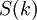$S(k)$, for a monatomic system is defined by: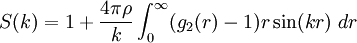$S(k) = 1 + \frac{4 \pi \rho}{k} \int_0^{\infty} ( g_2(r) -1 ) r \sin (kr) ~dr$

where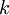$k$ is the scattering wave-vector modulus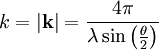$k= |\mathbf{k}|= \frac{4 \pi }{\lambda \sin \left( \frac{\theta}{2}\right)}$

The structure factor is basically a Fourier transform of the pair distribution function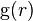${\rm g}(r)$,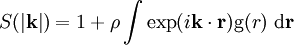$S(|\mathbf{k}|)= 1 + \rho \int \exp (i\mathbf{k}\cdot \mathbf{r}) \mathrm{g}(r) ~\mathrm{d}\mathbf{r}$

At zero wavenumber, i.e.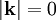$|\mathbf{k}|=0$,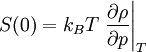$S(0) = k_BT \left. \frac{\partial \rho}{\partial p}\right\vert_T$

from which one can calculate the isothermal compressibility.

To calculate$S(k)$ in molecular simulations one typically uses: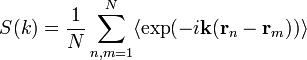$S(k) = \frac{1}{N} \sum^{N}_{n,m=1} \langle\exp(-i\mathbf{k}(\mathbf{r}_n-\mathbf{r}_m)) \rangle$,

where$N$ is the number of particles and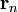$\mathbf{r}_n$ and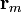$\mathbf{r}_m$ are the coordinates of particles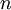$n$ and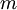$m$ respectively.

The dynamic, time dependent structure factor is defined as follows: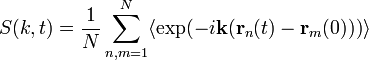$S(k,t) = \frac{1}{N} \sum^{N}_{n,m=1} \langle \exp(-i\mathbf{k}(\mathbf{r}_n(t)-\mathbf{r}_m(0))) \rangle$,

The ratio between the dynamic and the static structure factor,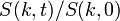$S(k,t)/S(k,0)$, is known as the collective (or coherent) intermediate scattering function.